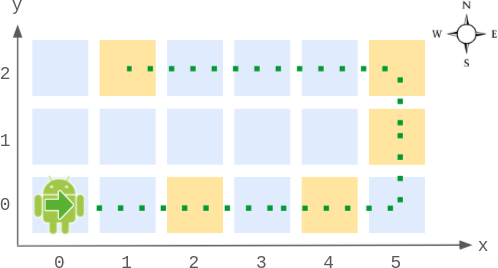# Walking Robot Simulation II solution leetcode

Contents

## Walking Robot Simulation II solution leetcode

`width x height` grid is on an XY-plane with the bottom-left cell at `(0, 0)` and the top-right cell at `(width - 1, height - 1)`. The grid is aligned with the four cardinal directions (`"North"``"East"``"South"`, and `"West"`). A robot is initially at cell `(0, 0)` facing direction `"East"`.

The robot can be instructed to move for a specific number of steps. For each step, it does the following. Walking Robot Simulation II solution leetcode

1. Attempts to move forward one cell in the direction it is facing.
2. If the cell the robot is moving to is out of bounds, the robot instead turns 90 degrees counterclockwise and retries the step.

After the robot finishes moving the number of steps required, it stops and awaits the next instruction.

Implement the `Robot` class:

• `Robot(int width, int height)` Initializes the `width x height` grid with the robot at `(0, 0)` facing `"East"`.
• `void move(int num)` Instructs the robot to move forward `num` steps.
• `int[] getPos()` Returns the current cell the robot is at, as an array of length 2, `[x, y]`.
• `String getDir()` Returns the current direction of the robot, `"North"``"East"``"South"`, or `"West"`.

Example 1: Walking Robot Simulation II solution leetcode```Input
["Robot", "move", "move", "getPos", "getDir", "move", "move", "move", "getPos", "getDir"]
[[6, 3], , , [], [], , , , [], []]
Output
[null, null, null, [4, 0], "East", null, null, null, [1, 2], "West"]

Explanation
Robot robot = new Robot(6, 3); // Initialize the grid and the robot at (0, 0) facing East.
robot.move(2);  // It moves two steps East to (2, 0), and faces East.
robot.move(2);  // It moves two steps East to (4, 0), and faces East.
robot.getPos(); // return [4, 0]
robot.getDir(); // return "East"
robot.move(2);  // It moves one step East to (5, 0), and faces East.
// Moving the next step East would be out of bounds, so it turns and faces North.
// Then, it moves one step North to (5, 1), and faces North.
robot.move(1);  // It moves one step North to (5, 2), and faces North (not West).
robot.move(4);  // Moving the next step North would be out of bounds, so it turns and faces West.
// Then, it moves four steps West to (1, 2), and faces West.
robot.getPos(); // return [1, 2]
robot.getDir(); // return "West"

```

Constraints: Walking Robot Simulation II solution leetcode

• `2 <= width, height <= 100`
• `1 <= num <= 105`
• At most `104` calls in total will be made to `move``getPos`, and `getDir`.